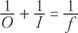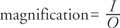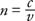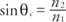## Geometrical Optics

When an object is dropped in still water, the circular wave fronts that are produced move out from the contact point over the two‐dimensional surface. A light source emits light uniformly in all directions of the three‐dimensional world. The wave fronts are spherical, and the direction of motion of the wave is perpendicular to the wave front, as depicted in Figure . This straight line path shown by the arrow is called a ray. Depicting light as rays in ray diagrams provides a method to explain the images formed by mirrors and lenses.Figure 1 Rays are perpendicular to the spherical wave fronts.

Far from the source, the curvature of the wave front is small, so the wave front appears to be a plane. Then, the light rays will be nearly parallel. Rays from the sun are considered to be parallel when reaching the earth.

## The law of reflection

Most visible objects are seen by reflected light. There are few natural sources of light, such as the sun, stars, and a flame; other sources are man‐made, such as electric lights. For an object to be visible, light from a source is reflected off the object into our eyes (except in the special case of phosphors). In Figure 2, the light is coming from the sun, parallel due to the distance of the source. The light reflects off the object and travels in straight lines to the viewer. Through experience, the viewer has learned to extend the reflected rays entering the eye back to locate the object.Figure 2

Vision is the result of light reflected from the object.

As shown in Figure 3, light strikes a mirror and is reflected. The original ray is called the incident ray, and after reflection, it is called the reflected ray. The angles of the incident and reflected rays are always measured from the normal. The normal is a line perpendicular to the surface at the point where the incident ray reflects. The incident ray, reflected ray, and normal all lie in the same plane perpendicular to the reflecting surface, known as the plane of incidence. The angle measured from the incoming ray to the normal is termed the incident angle. The angle measured from the outgoing ray to the normal is called the reflected angle. The law of reflection states that the angle of incidence equals the angle of reflection. This law applies to all reflecting surfaces.Figure 3 The law of reflection.

Light undergoes either diffuse or regular reflection. The two are illustrated in Figure 4.Figure 4 (a) Regular reflection. (b) Diffuse reflection.

Diffuse reflection occurs when light reflects from a rough surface. Regular reflection is reflection from a smooth surface, such as a mirror. The reflected rays are scattered in diffuse reflection. This scattering is because the local direction of the normal to the surface is different for the different rays. By contrast, in regular reflection, the reflected light rays are orderly because each local region of the surface has a normal in the same direction.

## Plane mirrors

Figure 5 illustrates the formation of an image by a plane mirror. Light rays are coming from a source and reflecting off each point of the object ( AB) in all directions. For simplicity, only a few of the rays are drawn. The rays spread upon leaving the object, and then each ray reflects from the mirror according to the law of reflection. The eye extends back the diverging reflected rays to see an image behind the mirror. An image formed in this manner by extending back the reflected diverging rays is called a virtual image. A virtual image cannot be projected on a screen. The light does not physically come together, but rather, the eye (or camera) interprets the diverging rays as originating from an image behind the mirror. Due to the law of reflection, the image formed by a plane mirror is the same distance behind the mirror as the object is in front of the mirror.Figure 5 Construction of an image reflected in a mirror.

How tall does a mirror need to be so you can see your entire height? Assume the top of the mirror is in line with the top of your head. Does it matter where you stand? The ray diagram in Figure illustrates this situation. From the law of reflection and basic geometry, it can be proven that the marked angles are all equal; therefore, the necessary height of the mirror is approximately half your height. Draw a figure at a different distance to show that the distance from the person to the mirror does not change the result.Figure 6 Seeing your own feet in a mirror.

## Concave mirrors

Regular reflection occurs not only for plane (flat) mirrors but also for curved mirrors. Picture a series of plane mirrors arranged in a semicircle as shown in Figure 7. The incoming light is from a distant source and, therefore, is nearly parallel, as Figure 7(a) shows. After reflection, the light converges on a region. As the number of mirrors increases—Figure 7(b)—the converging region of the light beams decreases.Figure 7

A semicircular arrangement of mirrors focuses light in the region F.

A concave mirror reflects its light from the inner curved surface. The mirror can be a portion of a sphere, a cylinder, or shaped as a rotated parabolic curve. The light rays intersect after reflection at a common focus called the focal point ( F). The focal point is on the optical axis, the symmetry axis of the mirror. The distance f from focal point to the mirror is called the focal length. For a spherical mirror, the focal length is one‐half the radius of the sphere that defines the mirror. This distance c is called the radius of curvature, and the center of the sphere is denoted as C( c = 2 f). Figure 8 illustrates these definitions.Figure 8 A concave mirror with radius c and focal length f.

It is helpful to have a geometric system for locating an image formed by rays reflected from a curved mirror. Any reflected ray follows the law of reflection; however, certain rays have easily defined paths so that measuring angles and finding the normals are not necessary. Four of these rays are

• The ray directed parallel to the optical axis will reflect through F.
• The ray directed through F will reflect parallel to the optical axis.
• The ray directed to the center of the mirror will reflect at the same angle to the optical axis.
• The ray directed along the radius of the sphere will reflect back on itself.

Light rays are drawn for four different positions in Figure 9 : (a) far from F, (b) at nearly 2 F, (c) between F and 2 F, and (d) at F. It is only necessary to find the intersection of two reflected rays from a point on the object to define the corresponding point on the image. A third one can be used as a check. Sometimes one or another of the rays may be difficult to draw, and so choices can be made.Figure 9 Images from a concave mirror.

Notice that images are formed for the first three cases but not for the last one. No image is formed when the object is at the focal point or, alternatively, the image is formed at infinity and cannot be seen. The three images are all real images. Real images can be shown on a screen because the light physically comes together at a point in space. Note that real images are formed by light that converges after reflection. Also, real images are always inverted—upside down—with regard to the original object. In Figure , the light rays from the bottom of the object are not drawn. Light traveling along the optical axis will reflect back along the axis, and so if a point of the object is on the optical axis, the corresponding image point will also be on the optical axis.

The images formed can be characterized by size and placement. Let the distance from the object to the mirror be given by O. Then the image characteristics can be summarized as follows:

• If O > 2 F, the image is inverted, smaller, and located between F and 2 F.
• If O = 2 F (at C), the image is inverted, the same size as the object, and located at 2 F; that is, the distances of both the object and image to the mirror are equal.
• If 2 F < O < F, the image is inverted, larger than the object, and located >2 F. Light paths are reversible. If the object is placed in the position of its former image, the image will then be located where the object was originally; that is, the two will exchange positions.

Figure 10 shows the diagram for the case when the object is between the focal point ( F) and the mirror. In this case, a virtual image is formed because the reflected rays diverge from the surface of the mirror. The virtual image is upright, enlarged, and behind the mirror. Virtual images are never inverted.Figure 10 Formation of a virtual image in a concave mirror.

The following approximate mirror equation relates the distances from the object to the mirror ( O), the distance from the image to the mirror ( I), and the focal length ( f):The sign of f is positive if it is on the same side as the mirror (a concave mirror) and negative otherwise (convex mirror). Both O and I are positive in sign if they lie on the same side of the mirror as the incident light and negative if they lie on the opposite side.

The magnification is defined as the ratio of the image size to the object size. This ratio is the same as the ratio of the distances:Thus, a magnification of 10 × means the image seen is 10 times the size of the object when viewed without a magnifying device.

## Convex mirrors

The graphical technique for locating the image of a convex mirror is shown in Figure . For convex mirrors, the image on the opposite side of the mirror is virtual, and the images on the same side of the mirror are real. Figure shows a virtual, upright, and smaller image. In comparison to the virtual image of the concave mirror, the virtual image of the convex mirror is still upright, but it is diminished (smaller) instead of enlarged and on the opposite side of the mirror instead of the same side. Again, the virtual image is formed by extending back the reflected diverging rays.Figure 11 Formation of a virtual image in a concave mirror.

## The law of refraction

Refraction is the bending of light when the beam passes from one transparent medium into another. A transparent object allows the transmission of light, in contrast to an opaque object, which does not. Some of the light will also be reflected. The incident ray, reflected ray, normal, and refracted ray are shown in Figure 12.Figure 12 The law of refraction.

When Willebrod Snell (1580–1626) observed light traveling from air into another transparent material, he found a constant ratio of the sines of the angles measured from the normal to the light ray in the material:The constant ( n) is called the index of refraction and depends only upon the optical properties of the material. The index of refraction gives a measure of the amount of bending occurring when light travels from air into the material. It is a dimensionless number and can be located in tables of properties of materials. For example, the index of refraction of water is 1.33, and the index of refraction of crown glass varies from 1.50 to 1.62, depending upon the composition of the glass.

For the more general case of light traveling from medium 1 to medium 2, Snell's law can be written n 1 sin θ 1 = n 2 sin θ 2, where the subscripts 1 and 2 refer respectively to the angles and indices of the refraction for material 1 and material 2 respectively. A light ray traveling along the normal, with an incident angle of zero, will not be bent.

The index of refraction is also the ratio of the speed of light in a vacuum ( c) and the speed of light in that medium ( v); thus,Consider the following problem involving both reflection and refraction. Imagine light entering an aquarium and reflecting off a mirror at the bottom. First, what will be the angle of refraction in the water if the angle in air is 30 degrees? Second, at what angle will the beam leave the water? See the setup in Figure 13.Figure 13 A problem combining refraction and reflection.

Angle θ 2 is determined from θ 1, using Snell's law of refraction. Angle θ 2 = θ 2 by geometry, θ 3 = θ 4 by law of reflection, and θ 4 = θ 5 by geometry. θ 6 is related to θ 5 by Snell's law of refraction, in the same ration as θ 1 to θ 2. Therefore, θ 6—the angle of the ray leaving the water—must be 30 degrees. The problem is symmetrical.

A light ray passing through a rectangular block of transparent material will simply be displaced from its original path. For example, in passing from air to glass, the ray will bend toward the normal. Upon leaving the glass block, the ray will bend away from the normal so that the measured angles in the air on each side of the block are the same (Figure 14).Figure 14 A light ray is displaced after passing through a refracting medium.

## Brewster's angle

Light reflected from the surface of a material is partially polarized. A ray incident on a transparent surface at a certain angle will be partly refracted and partly reflected in a plane polarized ray. This angle of maximum plane polarization is called Brewster's angle, named for Sir David Brewster (1781–1868). The equation is tan θ = n, where n is the index of refraction of the reflecting surface.

## Total internal reflection

When light travels from a material with a higher n to one with a lower n, at certain angles all of the light is reflected. This effect is called total internal reflection.

Example 1: Figure 15 illustrates ray 1 along the normal (no bending), rays 2 and 3 are refracted, and rays 5 and 6 are reflected. Ray 4 is intermediate between reflection and refraction with an angle of refraction of 90 degrees. The incident angle for this case is called the critical angle (θ). If the angle of incidence is less than θ, the light will refract, and if it is greater, the light will reflect.Figure 15

Total internal reflection at the interface of two different media.

The equation iswhere n 1 > n 2. Find the critical angle from glass to air.Therefore, if the incident ray on a glass to air interface is greater than 42 degrees, total internal reflection will occur. Figure 16 shows the light rays entering and leaving a 45‐45‐90 glass prism. This phenomenon has broad applications where a mirror is needed, but a silvered surface might corrode after a period of time.Figure 16 Total internal reflection in a glass prism.

## Optical lenses

An optical lens functions by refracting light at its interfaces. In these examples, the lens will be assumed to be thin, in which case the thickness of the lens is negligible compared with it focal length. Lenses are basically of two types. A converging lens causes parallel rays to converge, and a diverging lens causes parallel rays to diverge. Figure 17 illustrates the paths of the rays through the lens and the focal point for each case. The definitions for optical axis, focal point, and focal length given for curved mirrors hold true for lenses, with the addition that lenses have focal points on each side of the lens.Figure 17 Thin lenses function by refracting light.

Ray diagrams can be made for lenses similar to those drawn for curved mirrors. These three rays can be drawn to locate the image formed by the lens.

• The ray directed parallel to the optical axis refracts through F on the far side.
• The ray directed to the near F refracts parallel to the optical axis.
• The ray directed to the center of the lens is undeviated (in the thin lens approximation).
The ray diagrams for two cases of a converging lens are shown in Figure 18.Figure 18 Ray diagrams for a converging lens, showing the formation of (a) a real image or (b) a virtual image.

In Figure 18(a), a real image is formed, and in Figure 18(b), a virtual image is formed. The lens setup in Figure 18(b) is called a simple magnifier. With lenses as with mirrors, virtual images are right side up, and real images are inverted. (This is why slides inserted into a projector are inverted; the projector lens reinverts the image on the screen.)

The lens equation is the same relationship used for curved mirrors:as is the equation for magnification:The focal length is positive for a converging lens and negative for a diverging lens. The object and image distances are positive if they are on opposite sides of the lens and negative if they are on the same side. The relative sizes and positions of the object and image for a converging lens are similar to the four cases reviewed for the concave mirror.

• If O > 2 F, the image is inverted, smaller, and located between F and 2 F, on the opposite side.
• If O = 2 F, the image is inverted, the same size as the object, and located at 2 F; that is, the distances of both the object and image to the lens are equal but on opposite sides of the lens.
• If 2 F < O < F, the image is inverted, larger than the object, and located > 2 F.
• If O < F, the image is virtual, enlarged, and located on the same side of the lens where I > F.

Figure 19 shows the ray diagram for a diverging lens. The image formed by this lens is always virtual, upright, and diminished.Figure 19 Ray diagram for a diverging lens.

## The compound microscope

When lenses are used in combinations, the image given by one lens becomes the object for a second lens. The compound microscope is an example of the use of several lenses to magnify an object. An objective lens near the object forms an enlarged image. This image is then further magnified by the second lens, called the eyepiece. Both are converging lenses.

In Figure 20, the object ( AB) is placed just below the focal point of the objective lens. The objective lens forms an enlarged, real, and inverted image at a distance greater than 2 F from the first lens. This image ( AB′) falls inside the focal point of the eyepiece lens; therefore, an enlarged, virtual image is formed by the eyepiece ( AB″). The total magnification is the product of the magnifications of each lens.Figure 20 A compound microscope.

## Dispersion and prisms

An important property of the index of refraction is that it is slightly dependent upon wavelength. For a given material—for example, glass— n decreases with increasing wavelength; thus, blue light bends more than red light. This effect is called dispersion.

Light is refracted twice as it enters and leaves the prism, as shown in Figure 21.Figure 21 Dispersion of white light in a prism.

A given ray is bent from its original direction of travel by an angle (δ), called the angle of deviation. The angle of deviation for the red light is less than that for the blue light; therefore, the prism spreads the light into the colors of the spectrum. These colors are commonly called red, orange, yellow, green, blue, indigo, and violet (often abbreviated with the mnemonic Roy G Biv).

Rainbows are formed by dispersion and total internal reflection of sunlight in raindrops. The critical angle for water to air is approximately 40 degrees. The sunlight enters the drop and is reflected off the side of the drop away from the viewer. Due to dispersion, the violet ray emerges above the red ray. Figure 22 shows the refraction of sunlight on one idealized raindrop.Figure 22

Dispersion and internal reflection of light passing through a raindrop.

Figure 23 shows how the viewer sees the rainbow. The rainbow is in the shape of an arc because the circle of drops at the angle of about 40 degrees is in existence only above the ground. It is possible to see a circular rainbow from an airplane in the correct position relative to the sunlight and raindrops.Figure 23 Formation of a rainbow due to total internal reflection and dispersion.